JMSLTM Numerical Library 7.2.0
com.imsl.stat

## Class ClusterKMeans

• All Implemented Interfaces:
Serializable, Cloneable

```public class ClusterKMeans
extends Object
implements Serializable, Cloneable```
Perform a K-means (centroid) cluster analysis.

`ClusterKMeans` is an implementation of Algorithm AS 136 by Hartigan and Wong (1979). It computes K-means (centroid) Euclidean metric clusters for an input matrix starting with initial estimates of the K cluster means. It allows for missing values (coded as NaN, not a number) and for weights and frequencies.

Let p denote the number of variables to be used in computing the Euclidean distance between observations. The idea in K-means cluster analysis is to find a clustering (or grouping) of the observations so as to minimize the total within-cluster sums of squares. In this case, the total sums of squares within each cluster is computed as the sum of the centered sum of squares over all nonmissing values of each variable. That is,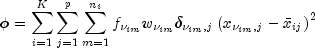where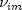denotes the row index of the m-th observation in the i-th cluster in the matrix X;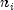is the number of rows of X assigned to group i; f denotes the frequency of the observation; w denotes its weight; d is zero if the j-th variable on observationis missing, otherwise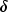is one; and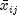is the average of the nonmissing observations for variable j in group i. This method sequentially processes each observation and reassigns it to another cluster if doing so results in a decrease in the total within-cluster sums of squares. See Hartigan and Wong (1979) or Hartigan (1975) for details.

ClusterKMeans Example, Serialized Form
• ### Nested Class Summary

Nested Classes
Modifier and Type Class and Description
`static class ` `ClusterKMeans.ClusterNoPointsException`
There is a cluster with no points
`static class ` `ClusterKMeans.NoConvergenceException`
Convergence did not occur within the maximum number of iterations.
• ### Constructor Summary

Constructors
Constructor and Description
```ClusterKMeans(double[][] x, double[][] cs)```
Constructor for `ClusterKMeans`.
• ### Method Summary

Methods
Modifier and Type Method and Description
`double[][]` `compute()`
Computes the cluster means.
`int[]` `getClusterCounts()`
Returns the number of observations in each cluster.
`int[]` `getClusterMembership()`
Returns the cluster membership for each observation.
`double[]` `getClusterSSQ()`
Returns the within sum of squares for each cluster.
`void` `setFrequencies(double[] frequencies)`
Sets the frequency for each observation.
`void` `setMaxIterations(int iterations)`
Sets the maximum number of iterations.
`void` `setWeights(double[] weights)`
Sets the weight for each observation.
• ### Methods inherited from class java.lang.Object

`clone, equals, finalize, getClass, hashCode, notify, notifyAll, toString, wait, wait, wait`
• ### Constructor Detail

• #### ClusterKMeans

```public ClusterKMeans(double[][] x,
double[][] cs)```
Constructor for `ClusterKMeans`.
Parameters:
`x` - A `double` matrix containing the observations to be clustered.
`cs` - A `double` matrix containing the cluster seeds, i.e. estimates for the cluster centers.
• ### Method Detail

• #### compute

```public final double[][] compute()
throws ClusterKMeans.NoConvergenceException,
ClusterKMeans.ClusterNoPointsException```
Computes the cluster means.
Returns:
A `double` matrix containing computed result.
Throws:
`com.imsl.stat.ClusterKMeans.NonnegativeFreqException` - is thrown if a frequency is negative.
`com.imsl.stat.ClusterKMeans.NonnegativeWeightException` - is thrown if a weight is negative.
`ClusterKMeans.NoConvergenceException` - is thrown if convergence did not occur within the maximum number of iterations.
`ClusterKMeans.ClusterNoPointsException` - is thrown if the cluster seed yields a cluster with no points.
• #### getClusterCounts

`public int[] getClusterCounts()`
Returns the number of observations in each cluster. Note that the `compute` method must be invoked first before invoking this method. Otherwise, the method throws a `NullPointerException` exception.
Returns:
An `int` array containing the number of observations in each cluster.
• #### getClusterMembership

`public int[] getClusterMembership()`
Returns the cluster membership for each observation. Note that the `compute` method must be invoked first before invoking this method. Otherwise, the method throws a `NullPointerException` exception.
Returns:
An `int` array containing the cluster membership for each observation. Cluster membership 1 indicates the observation belongs to cluster 1, cluster membership 2 indicates the observation belongs to cluster 2, etc.

`public double[] getClusterSSQ()`
Returns the within sum of squares for each cluster. Note that the `compute` method must be invoked first before invoking this method. Otherwise, the method throws a `NullPointerException` exception.
Returns:
A `double` array containing the within sum of squares for each cluster.
• #### setFrequencies

`public void setFrequencies(double[] frequencies)`
Sets the frequency for each observation.
Parameters:
`frequencies` - A `double` array of size `x.length` containing the frequency for each observation. Default: `frequencies[]` = 1.
• #### setMaxIterations

`public void setMaxIterations(int iterations)`
Sets the maximum number of iterations.
Parameters:
`iterations` - An `int` scalar specifying the maximum number of iterations. Default: `interations` = 30.
• #### setWeights

`public void setWeights(double[] weights)`
Sets the weight for each observation.
Parameters:
`weights` - A `double` array of size `x.length` containing the weight for each observation. Default: `weights[]` = 1.
JMSLTM Numerical Library 7.2.0